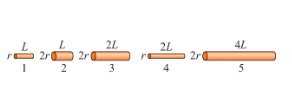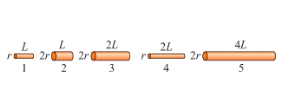# Problem: The wires in the figure are all made of the same material; the length and radius of each wire is noted.Rank in order, from largest to smallest, the resistances R1 to R5 of these wires. Rank from largest to smallest. To rank items as equivalent, overlap them.R1, R2, R3, R4, R5

###### FREE Expert Solution

R = ρL/A

For circular wires,

R = ρL/(πr2)L doubles → 2x resistance

r doubles → 4x resistance

91% (307 ratings)###### Problem Details

The wires in the figure are all made of the same material; the length and radius of each wire is noted.Rank in order, from largest to smallest, the resistances R1 to R5 of these wires. Rank from largest to smallest. To rank items as equivalent, overlap them.

R1, R2, R3, R4, R5